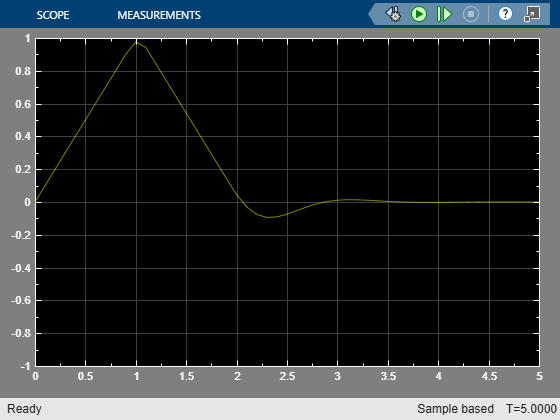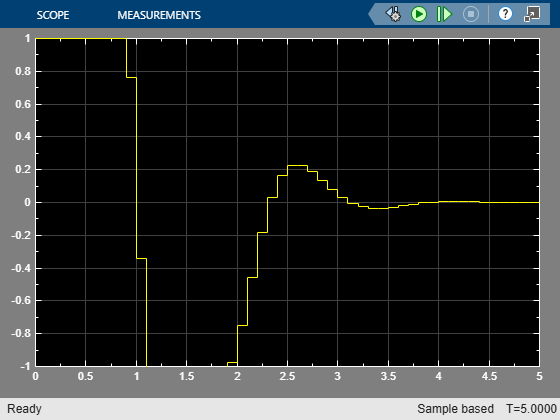Documentation

## Understanding Control Behavior by Examining Optimal Control Sequence

This example shows how to inspect the optimized sequence of manipulated variables computed by a model predictive controller at each sample time.

The plant is a double integrator subject to input saturation.

### Design MPC Controller

The basic setup of the MPC controller includes:

• A double integrator as the prediction model

• Prediction horizon of `20`

• Control horizon of `10`

• Input constraints `-1 <= u(t) <= 1`

Configure the MPC controller.

```Ts = 0.1; p = 20; m = 10; mpcobj = mpc(tf(1,[1 0 0]),Ts,p,m); mpcobj.MV = struct('Min',-1,'Max',1); nu = 1; ```
```-->The "Weights.ManipulatedVariables" property of "mpc" object is empty. Assuming default 0.00000. -->The "Weights.ManipulatedVariablesRate" property of "mpc" object is empty. Assuming default 0.10000. -->The "Weights.OutputVariables" property of "mpc" object is empty. Assuming default 1.00000. ```

To run this example, Simulink® is required.

```if ~mpcchecktoolboxinstalled('simulink') disp('Simulink is required to run this example.') return end ```

Open the Simulink model, and run the simulation.

```mdl = 'mpc_sequence'; open_system(mdl) sim(mdl) ```
```-->Converting the "Model.Plant" property of "mpc" object to state-space. -->Converting model to discrete time. Assuming no disturbance added to measured output channel #1. -->The "Model.Noise" property of the "mpc" object is empty. Assuming white noise on each measured output channel. ```The MPC Controller block has an `mv.seq` output port, which is enabled by selecting the Optimal control sequence block parameter. This port outputs the optimal control sequence computed by the controller at each sample time. The output signal is an array with `p`+1 rows and `Nmv` columns, where `p` is prediction horizon and `Nmv` is the number of manipulated variables.

In a similar manner, the controller can output the optimal state sequence (`x.seq`) and the optimal output sequence (`y.seq`).

The model exports this control sequence to the MATLAB® workspace at each simulation step, logging the data as `useq`.

### Analyze Optimal Control Sequences

Plot the optimal control sequence at specific time instants.

```times = [0 0.2 1 2 2.1 2.2 3 3.5 5]; figure('Name','Optimal sequence history') for t = 1:9 ct = times(t)*10+1; subplot(3,3,t) h = stairs(0:p,useq.signals.values(ct,:)); h.LineWidth = 1.5; hold on plot((0:p)+.5,useq.signals.values(ct,:),'*r') xlabel('prediction step') ylabel('u') title(sprintf('Sequence (t=%3.1f)',useq.time(ct))) grid axis([0 p -1.1 1.1]) hold off end ```The MPC controller uses the first two seconds to bring the output very close to the new set point. The controller output is at the high limit (+1) for one second and switches to the low limit (-1) for the next second, which is the best control strategy under the input constraint limits.

```bdclose(mdl) ```